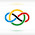### 2005 IMO SL #C7

Suppose that $a_1$, $a_2$, $\ldots$, $a_n$ are integers such that $n\mid a_1 + a_2 + \ldots + a_n$. Prove that there exist two permutations $\left(b_1,b_2,\ldots,b_n\right)$ and $\left(c_1,c_2,\ldots,c_n\right)$ of $\left(1,2,\ldots,n\right)$ such that for each integer $i$ with $1\leq i\leq n$, we have $n\mid a_i - b_i - c_i$

Suppose there exist permutations $\sigma$ and $\tau$ of $[n]$ for some sequence $\{ a_i\}_{i\in [n]}$ so that $a_i\equiv_n \sigma (i)+\tau (i)$ for all $i\in [n]$. Given a sequence $\{ b_i\}_{i\in [n]}$ with sum divisible by $n$ that differ, in modulo $n$, from $\{ a_i\}_{i\in [n]}$ in only two positions, say $i_1$ and $i_2$. We wish to construct permutations $\sigma'$ and $\tau'$ of $[n]$ such that $b_i\equiv_n \sigma' (i) +\tau' (i)$ for all $i\in [n]$. Recall that $b_i\equiv a_i\pmod{n}$ for all $i\in [n]$ that $i\neq i_1,i_2$. Consider a sequence $i_1,i_2,i_3,...$ by, for each integer $k\geq 2$, define $i_{k+1}\in [n]$ to be the unique integer satisfy $\sigma (i_{k-1})+\tau (i_{k+1})\equiv_n b_{i_k}$. Let (clearly exists) $p<q$ are the indices that $i_p=i_q$ with minimal $p$, and then minimal $q$. If $p>2$, then $i_j\not\in \{ i_1,i_2\} \implies \sigma (i_j) +\tau (i_j) \equiv_n b_{i_j}$ for all $j\in \{ p,p+1,...,q\}$. Summing the equation $\sigma (i_{k-1})+\tau (i_{k+1})\equiv_n b_{i_k}$ for $k\in \{ p,p+1,...,q-1\}$ gives us $$\sum_{j=p-1}^{q-2}{\sigma (i_j) } +\sum_{j=p+1}^{q}{\tau (i_j)} \equiv_n\sum_{j=p}^{q-1}{b_{i_j}} \implies \sigma (i_{p-1}) +\sigma (i_p) +\tau (i_{q-1}) +\tau (i_q) \equiv_nb_{i_p}+b_{i_{q-1}}.$$Plugging $i_p=i_q$ and using the fact that $\sigma (i_p) +\tau (i_p)\equiv_n b_{i_p}$, we obtain $$\sigma (i_{p-1}) +\tau (i_{q-1})\equiv_n b_{i_{q-1}} \equiv_n \sigma (i_{q-1})+\tau (i_{q-1}).$$Hence, $\sigma (i_{p-1}) \equiv_n \sigma (i_{q-1})\implies i_{p-1}=i_{q-1}$, which is a contradiction to the definition of $p,q$. So, we know that $p\in \{ 1,2\}$. Let $p'=3-p$. Define the desired permutations $\sigma'$ and $\tau'$ as follows: $$\sigma' (i_l)=\begin{cases} \sigma (i_{l-1}), & \text{ if } l\in \{ 2,3,...,q-1\} \\ \sigma (i_{q-1}), & \text{ if } l=1 \end{cases} ,\tau' (i_l)= \begin{cases} \tau (i_{l+1}), & \text{ if } l\in \{ 2,3,...,q-1\} \\ \tau (i_{p'}), & \text{ if } l=1 \end{cases}$$and $\sigma' (i) =\sigma (i),\tau' (i)=\tau (i)$ for the rest $i\in [n]$ that $i\not\in \{ i_1,i_2,...,i_{q-1}\}$. Note that the reason we choose $\tau (i_{p'})$ is just to not use $\tau (i_p)=\tau (i_{(q-1)+1})$ more than once. This construction gives us $\sigma' (i)+\tau' (i)\equiv_n b_i$ for all $i\in [n]$ except when $i=i_1$. But since both $\sigma'$ and $\tau'$ are permutations of $[n]$, we have $$\sum_{i\in [n]}{(\sigma' (i)+\tau' (i))} \equiv_n 2\times \frac{n(n-1)}{2}\equiv_n 0\equiv_n \sum_{i\in [n]}{b_i}.$$ This guarantees that $\sigma' (i) +\tau' (i)\equiv_n b_i$ when $i=i_1$ too. So, this proves the validity of permutations we constructed.

We are done. $\square$

1.Anonymous3/04/2022

ah let's go new post

2.Anonymous3/07/2022

the subscription is great :)

now i get notified whenever there is a new post

3.Anonymous3/07/2022

by the way, just to get an idea, how often will you be posting going forward?

4.@Anonymous

I'm going to be posting one post every 3-4 days.

If this changes and I plan to post more frequently or less frequently (which it most likely won't), I will make a post about it.

5.Hello! :)

6.@Lukas

Hi,

Can you tell me what exactly you want to know about my routine, so that I can respond more specifically to your question?

7.Mate, I was just wondering how you manage your time and how exactly you are able to do these level of problems. Because I've not seen many 8th graders that do these challenging IMO problems.

8.@Lukas

This year, I had virtual school because of COVID so I got a lot of time to do this stuff.

As for the second part, luckily, I was exposed to these problems at an early stage (thanks to my dad 🙂).

9.I see.

I wish I had virtual school too :(

Noone in my family does math but I've been able to learn some math using aops.com.

Your blog has been helping me a lot for the past few months to learn IMO level of math competitions, because aops doesn't have much of that. I don't know what on earth you do with your solutions, but somehow they help me understand how to think of it myself rather than just memorizing the "trick". When I read other solutions, this doesn't happen.

Thank you for making this and touching lives :)

10.@Lukas

I'm glad it is helping you :D